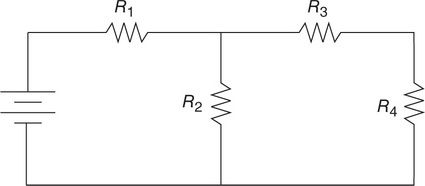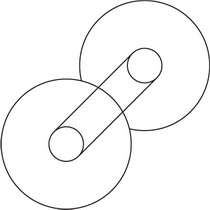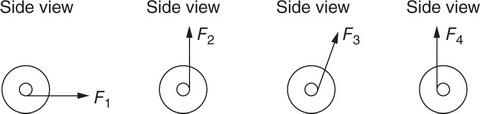# AP Physics 1 Practice Test 15

### Test Information10 questions18 minutes

1. Which of the following force diagrams could represent the forces acting on a block that slides to the right while slowing down?

2. Standing waves are produced by a 100-Hz generator in a string of fixed length. The tension in the string is increased until a new set of standing waves is produced. Will the wavelength of the new standing waves be greater than or less than the wavelength of the original standing waves?

3. Two electrically charged balls are separated by a short distance, producing a force of 50 μN between them. Keeping the charge of each ball the same, the mass of one of the balls but not the other is doubled. What is the new electric force between the balls?

4. A block of mass m is attached to a spring of force constant k. The mass is stretched a distance A from equilibrium and released from rest. At a distance x from the equilibrium position, which of the following represents the kinetic energy of the block?

5. A man stands with his hands to his sides on a frictionless platform that is rotating. Which of the following could change the angular momentum of the man-platform system?

6. The distance between the centers of two objects is d. Each object has identical mass m and identical charge –q. Choose all of the correct statements about the similarities and differences between the electric and gravitational force between the two objects. Select two answers.

7.Which placement of voltmeters will allow for determination of the voltage across resistor R2 in the circuit diagrammed in the preceding figure? Select two answers.

8. A student of mass 50 kg stands on a scale in an elevator. The scale reads 800 N. Which of the following could describe how the elevator is moving? Select two answers.

9. A 1-m-long pipe is closed at one end. The speed of sound in the pipe is 300 m/s. Which of the following frequencies will resonate in the pipe? Select two answers.

10.The device shown in the preceding figure consists of two wheels connected by a thick axle. A force can be applied to the axle by pulling a rope at several positions along the axle. Assuming the spool does not slip on the table, which of the pictured applied forces would cause rotation to the right of the device’s wheels? Select two answers.